Disclosure: This post may contain affiliate links, meaning when you click the links and make a purchase, we receive a commission.

# How to remove leading zeros in Excel (8 easy ways)

In this article, I will show you a good number of techniques on how to remove leading zeros in Excel. We don’t create all our worksheets ourselves. Sometimes we deal poorly formatted worksheets where you might find numbers are formatted as text. In text formats, numbers will show the leading zeros.

## How to remove leading zeros in Excel

### 1) Converting text to the number (the most convenient way)

This is the easiest way. Choose those cells that are showing numbers with leading zeros.

Click on the little yellow icon that appears when you select the cell range.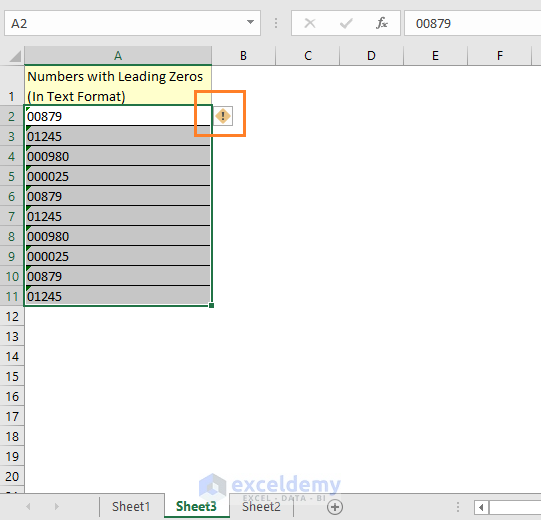A drop-down menu will appear with several options there. Choose Convert to Number option.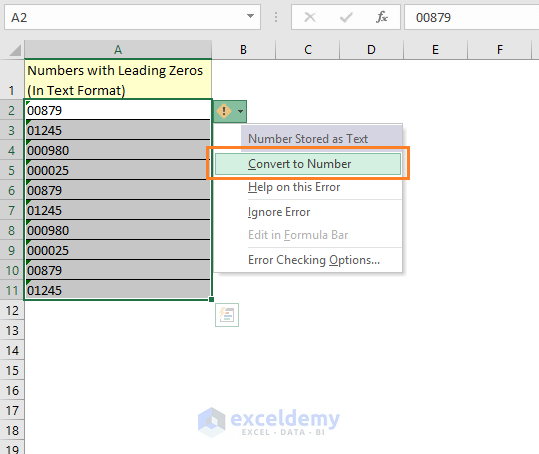You see all the numbers are converted to numbers and with no leading zeros.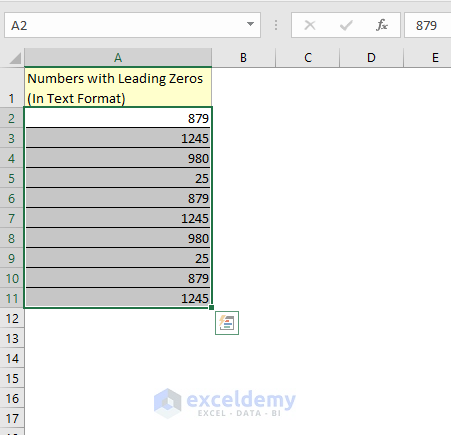In this article, you will learn a good number of techniques on how to convert text to numbers in Excel.

### 2) Using Excel’s VALUE () function

You can also use Excel’s VALUE function. If numbers are formatted as text and you use VALUE function on them, you will get the numbers.

Take a look at the following image, you will get the idea of how Excel VALUE function works.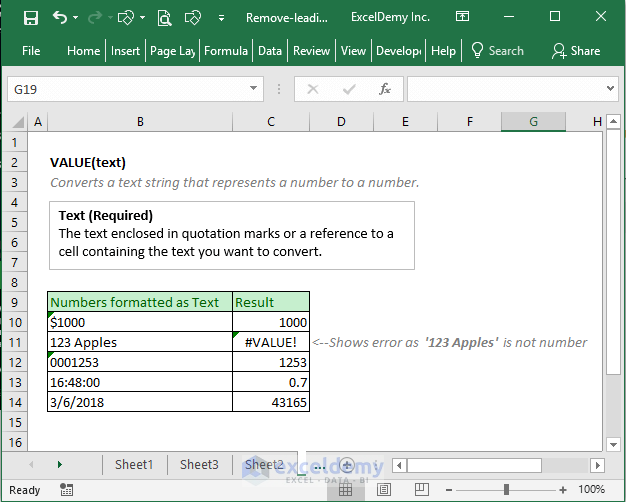Let’s now apply the VALUE function on our text formatted numbers with leading zeros.

I input a value formula in cell B2 as the following image.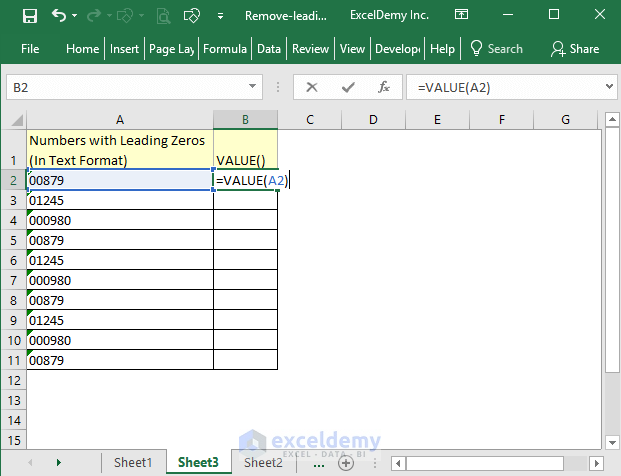I use the formula for all other cells in the column and here is the result.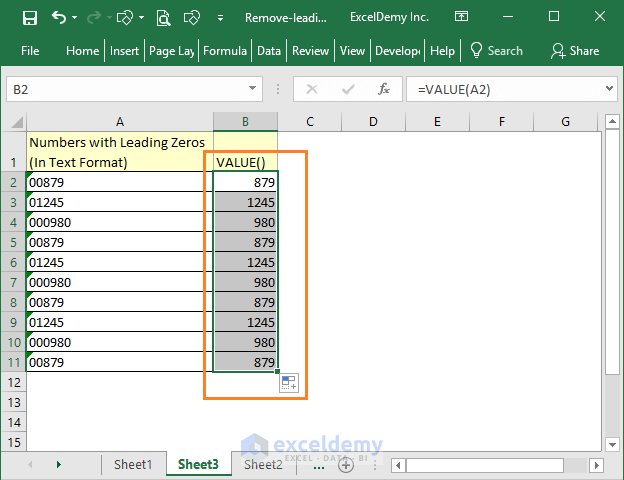### 3) Using VALUE and TEXT functions together

VALUE function will change the text formatted numbers to numbers, but what if you want to remove the leading zeros but want to keep the text formatted numbers in the text format. You have to use TEXT and VALUE functions together.

Use this formula in cell `B2: =TEXT(VALUE(A2), "#")`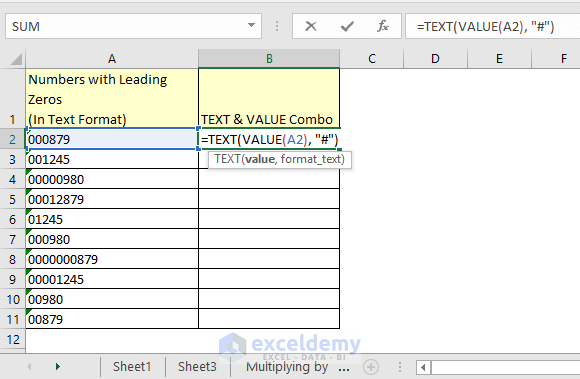You see the results here.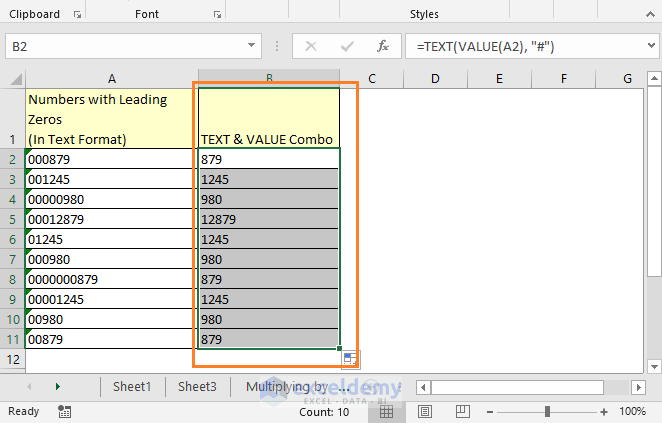VALUE function converts the Text formatted numbers into numbers, but TEXT function’s this argument “#” converts them texts again.

### 4) Multiplying the column with 1 (useful if you want to keep the numbers in text format)

Make a new column with a multiplying factor (in this case 1). And here is the result.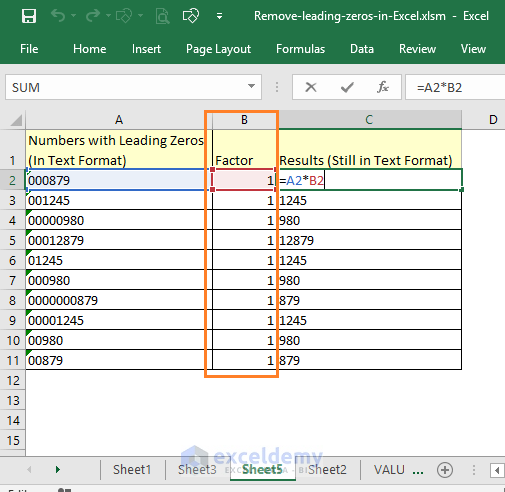Note: After using the formula for once, the cell will be formatted as text. So next time, no formulas will work on those cells. To keep formula working again, change the format of the cells to General.

### 5) Using Paste Special Command

You can also use Paste Special command to remove the leading zeros.

In a cell, say in cell C2, put 1 numeric value and copy the value. As shown in the following image.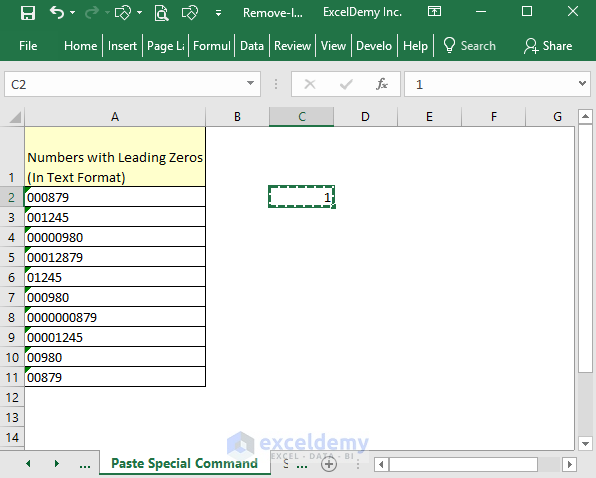Now select those cells that have the text formatted numbers => Right-click on the selection => And click on the Paste Special command.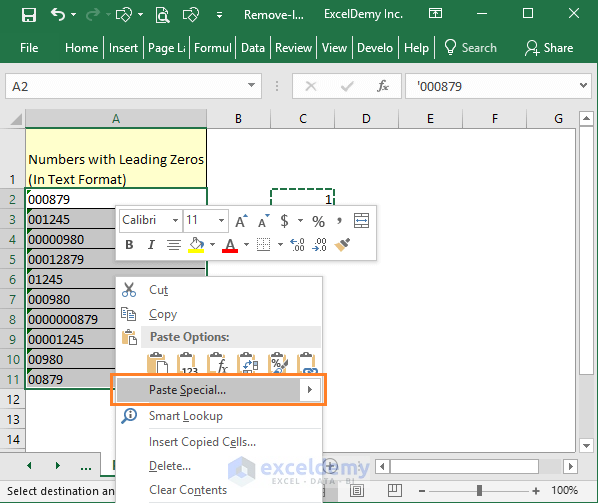Paste Special dialog box appears and choose Multiply option from this dialog box and then click OK.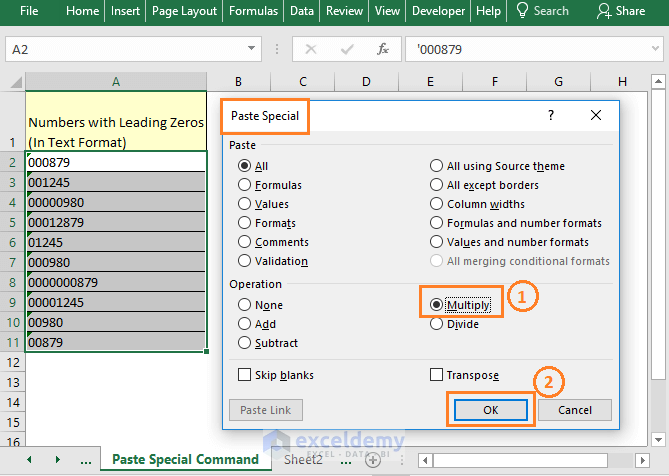And here is the result.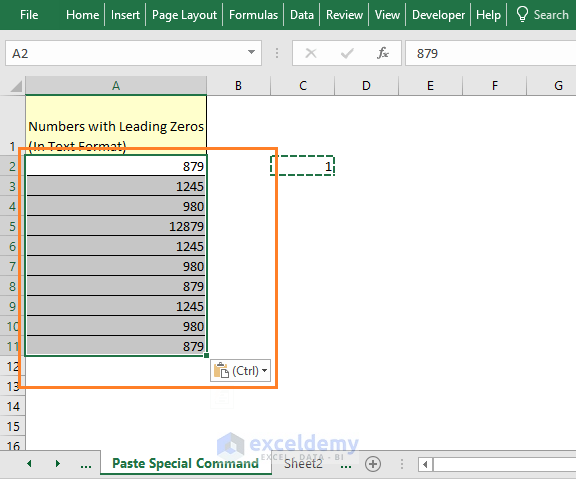### 6) Using Excel’s Text to Columns feature

We can also use Text to Columns feature in Excel to remove leading zeros from text-formatted numbers.

Select the cells => Data tab => Convert Text to Column Wizard opens => Click on Next.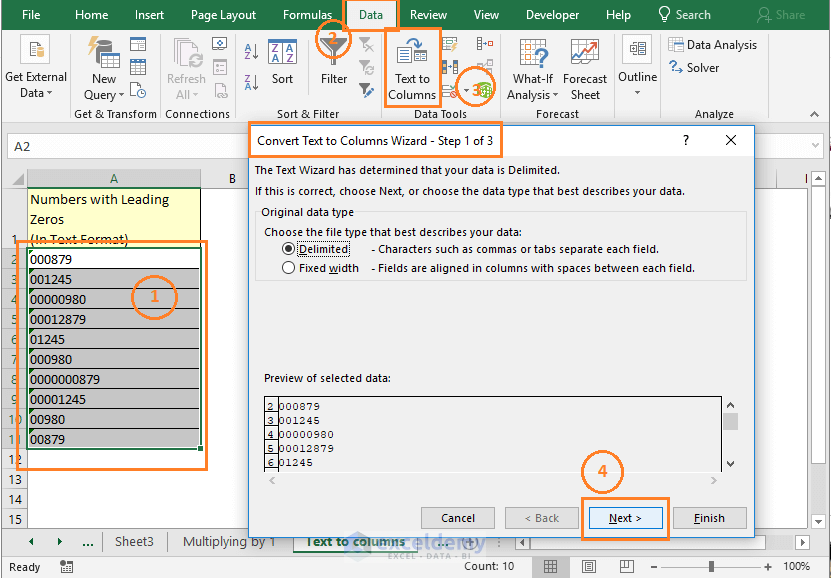As it is. Click Next again.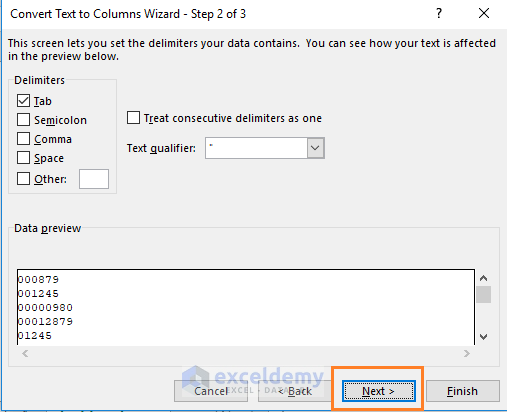I change the Destination cell in this step. And click on the Finish button.

I have changed the destination cell to another cell. If you want to replace the text formatted numbers with the numbers, keep the default Destination and it was \$A\$2.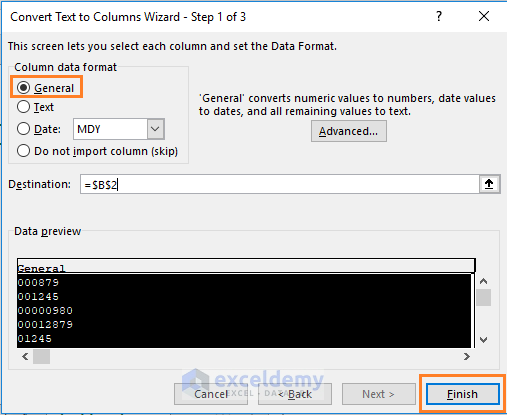And this is the result we get.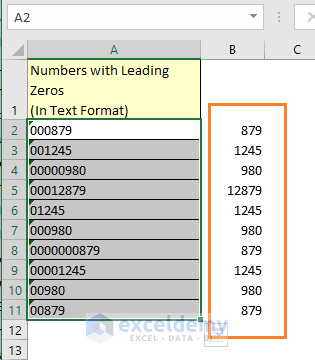### 7) Removing leading zeros from numbers with Excel VBA

I shall use a code to delete leading zeros from a cell or a range.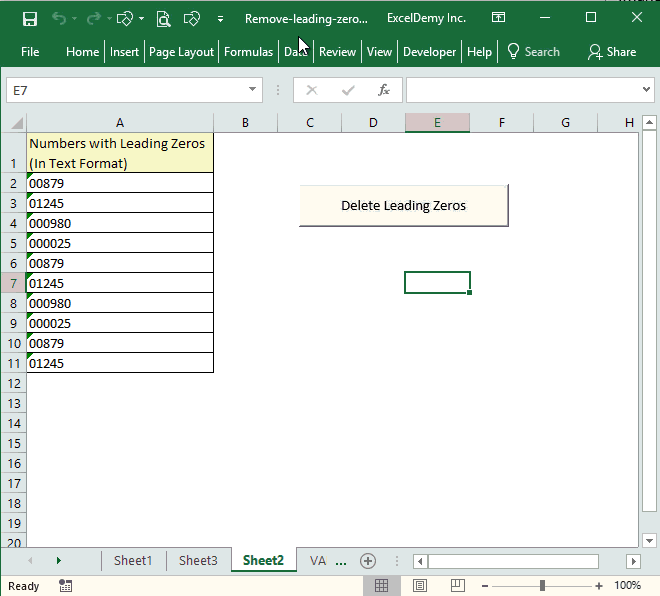This is the code that I have used to delete leading zeros from numbers.

 Source code```Sub Delete_Leading_Zero()
'Code by ExcelDemy.com
Dim Delete_Range As Range
Dim Work_Range As Range
On Error Resume Next
xTitleId = "ExcelDemy Delete Leading Zeros"
Set Work_Range = Application.Selection
Set Work_Range = Application.InputBox("Range", xTitleId, Work_Range.Address, Type:=8)
Work_Range.NumberFormat = "General"
Work_Range.Value = Work_Range.Value
End Sub```

#### # Where will you paste this code?

At first, open the Excel VBA editor. Developer Tab => Code window => Visual Basic command. Or just press ALT + F11 keys together on your keyboard to open the VBA editor.

And create a new Module from the Insert tab of the VBA Editor.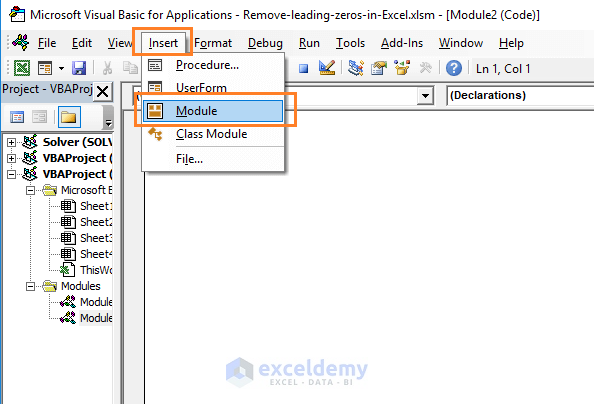Now copy-paste the above code in the Module.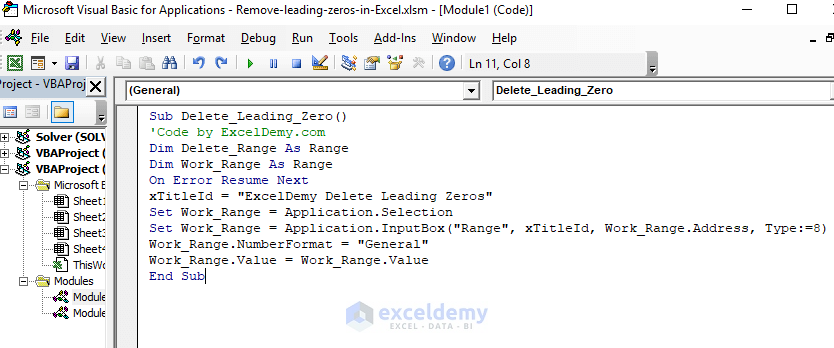#### # How to add this Macro to a Command Form Control?

Go to the Developer tab => Controls window => Click on the Insert drop-down => Choose the Button from the Form Controls part.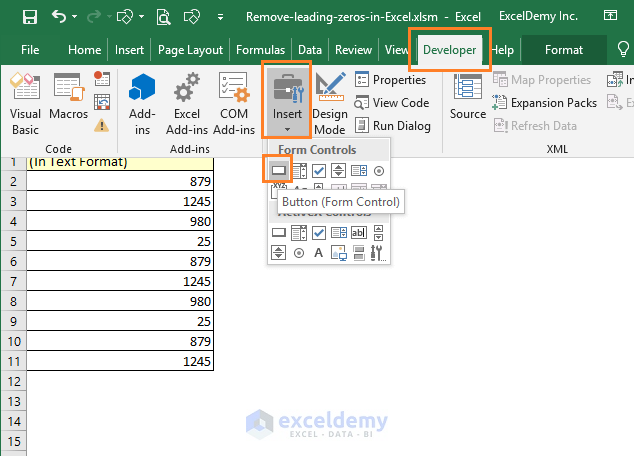Try to draw a button as shown in the image below.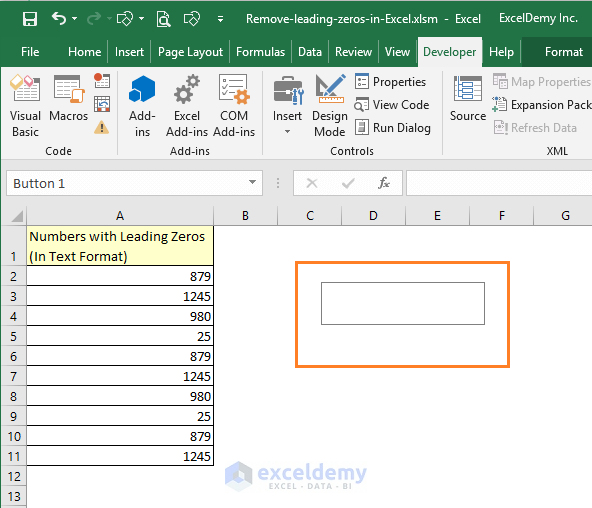Assign Macro dialog box will be available.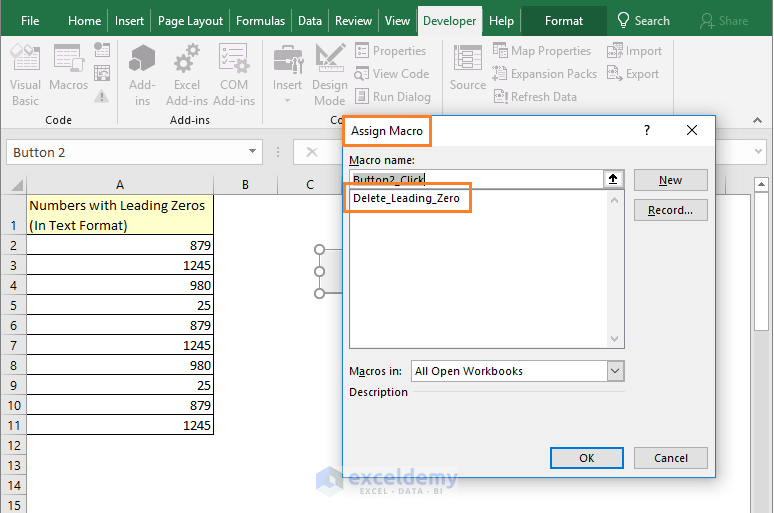In the dialog box, select the Delete_Leading_Zero macro for the button and click OK.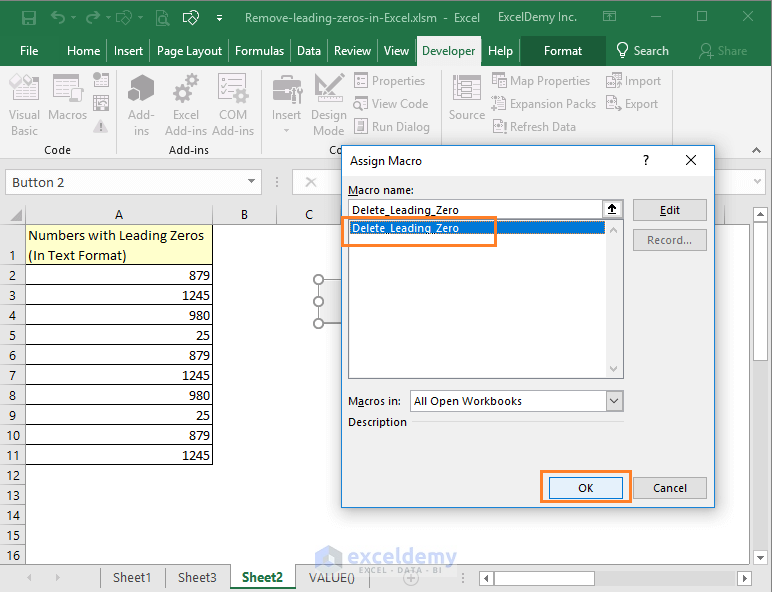By default, the command button will be named with a generic name. We just use a name suitable for the Macro.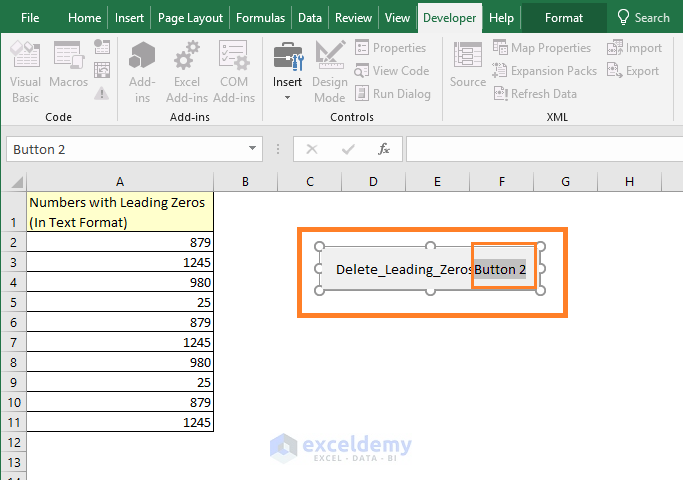This is the final thing.## How to remove leading zeros from non-numeric values?

This is a special case. See the following worksheet.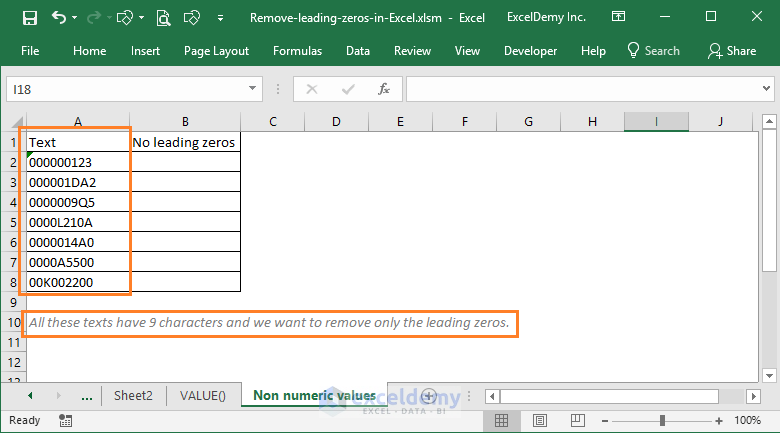All these texts have 9 characters and we want to remove only the leading zeros from these texts.

In cell B2, I use this formula:

=IF(LEFT(A2) = “0”, RIGHT(A2, LEN(A2)-1), A2)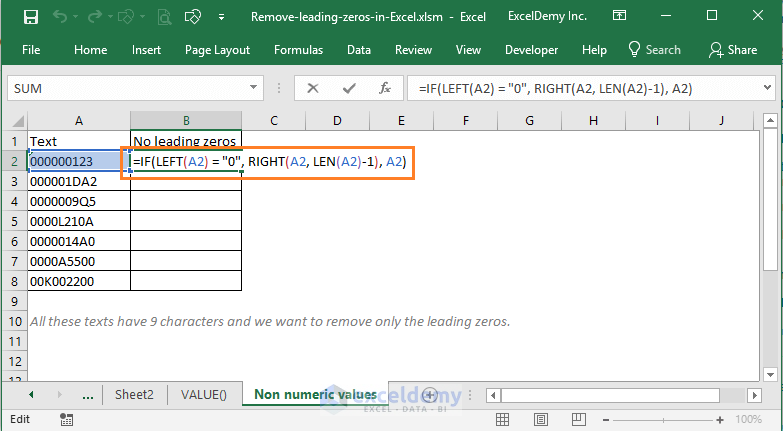This formula will remove the first leading zero from the text. To remove other leading zeros from this text, I extend this formula to the right-side cells (up to J column as at most 9 leading zeros can be in a text). And you see the results.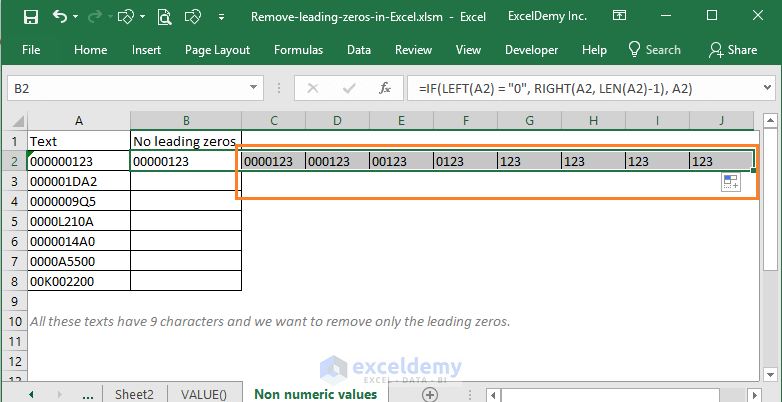Now just copy these formulas for other rows of the range. And you get your desired results at the last column of this range.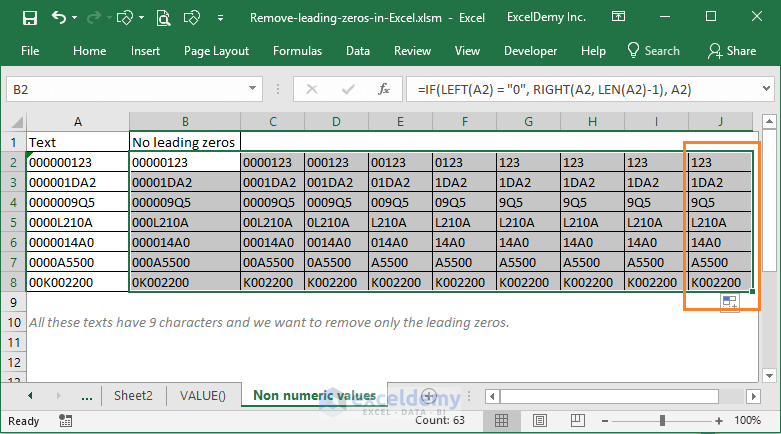## Conclusion

So, these are my ways of removing leading zeros from Excel numbers that are formatted as text. Do you know any other technique? Please let us know in the comment box.

Hello!
Welcome to my Excel blog! It took me some time to be a fan of Excel. But now I am a die-hard fan of MS Excel. I learn new ways of doing things with Excel and share here. Not only how to guide on Excel, but you will get also topics on Finance, Statistics, Data Analysis, and BI. Stay tuned!

1. ReplyFerreira Feb 24, 2019 at 11:16 PM

Very good, congratulations

• ReplyAug 17, 2020 at 11:40 PM

2. Reply• Reply